# Protractor

 1 The Geometry Box 2 Introduction to Protractor tool 3 What is Protractor tool? 4 How to use a Protractor? 5 Measuring Angles from Protractor 6 Draw angles with the Protractor 7 Important Notes on using Protractor 8 Solved Examples on Protractor 9 Common Mistakes 10 Challenging Questions on Protractor 11 Practice Questions on Protractor 12 Maths Olympiad Sample Papers 13 Frequently Asked Questions (FAQs)

We at Cuemath believe that Math is a life skill. Our Math Experts focus on the “Why” behind the “What.” Students can explore from a huge range of interactive worksheets, visuals, simulations, practice tests, and more to understand a concept in depth.

Book a FREE trial class today! and experience Cuemath's LIVE Online Class with your child.

## The Geometry Box

A geometry box consists of many tools like protractor, triangle protractor, compass etc.

We use a protractor to measure angles.

Architects and designers use a more precise protractor called an angle protractor tool which gives more accurate measures.

A compass helps to construct an angle.

Set squares, also known as triangle protractor, are used to draw parallel and perpendicular lines.

The divider is used to measure the length between two points.

## Introduction to Protractor Tool

Protractors have been in use since the seventeenth century. It is used for navigation in the sea and land.

Later by eighteenth century, protractors were widely used in geometry and mathematics.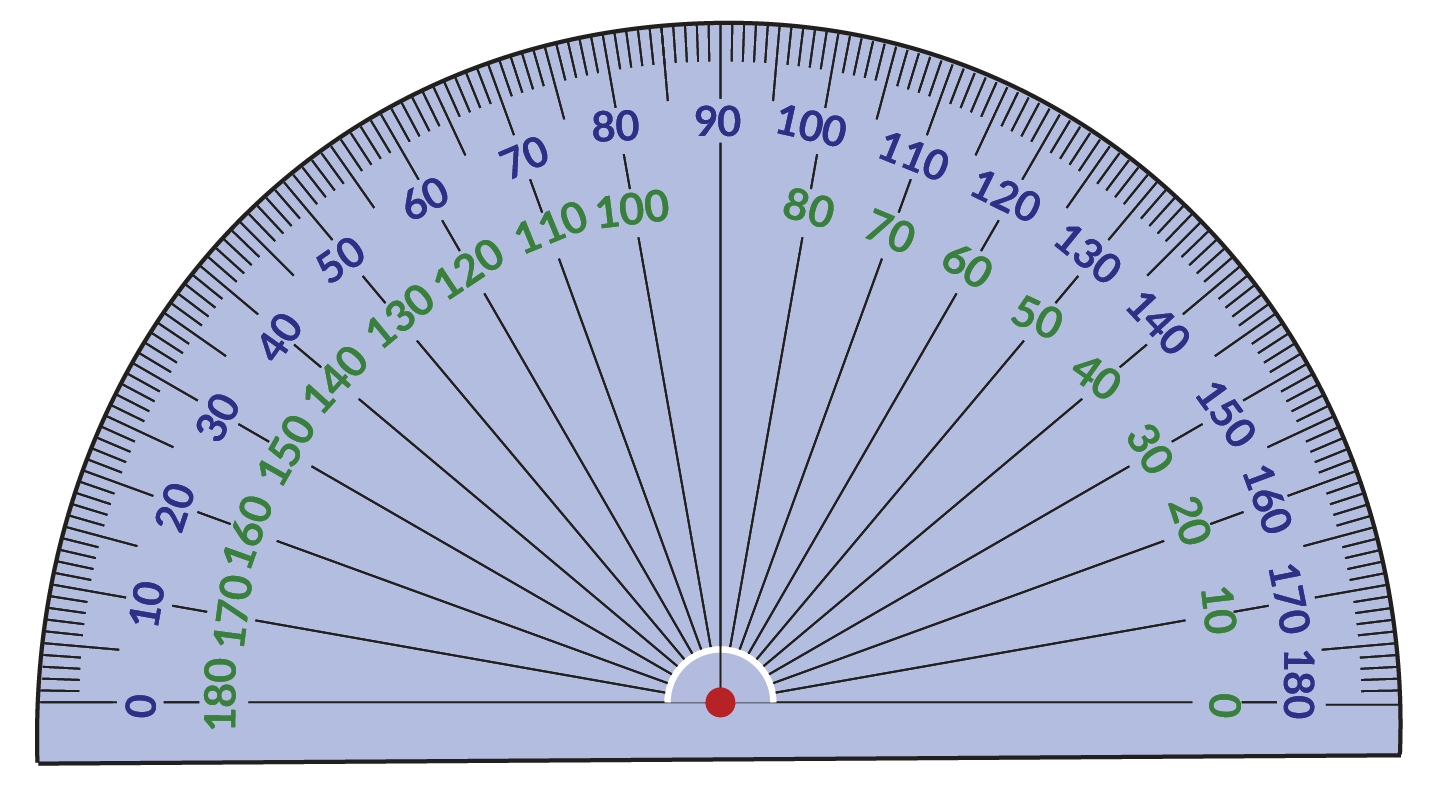### Protractor: Definition

The common protractor is a simple, measuring instrument that is typically semicircle in shape with markings from $$0 ^\circ$$ to $$180^\circ$$ on it.

## What is a Protractor Tool?

Protractor in maths is considered an important measuring instrument in the geometry box.

The protractor tool helps us measure an angle in degrees.

If you observe closely, a protractor tool has degrees marked from $$0$$ to $$180$$ from left to right in the outer edge and from$$180$$ to $$0$$ in the inner part.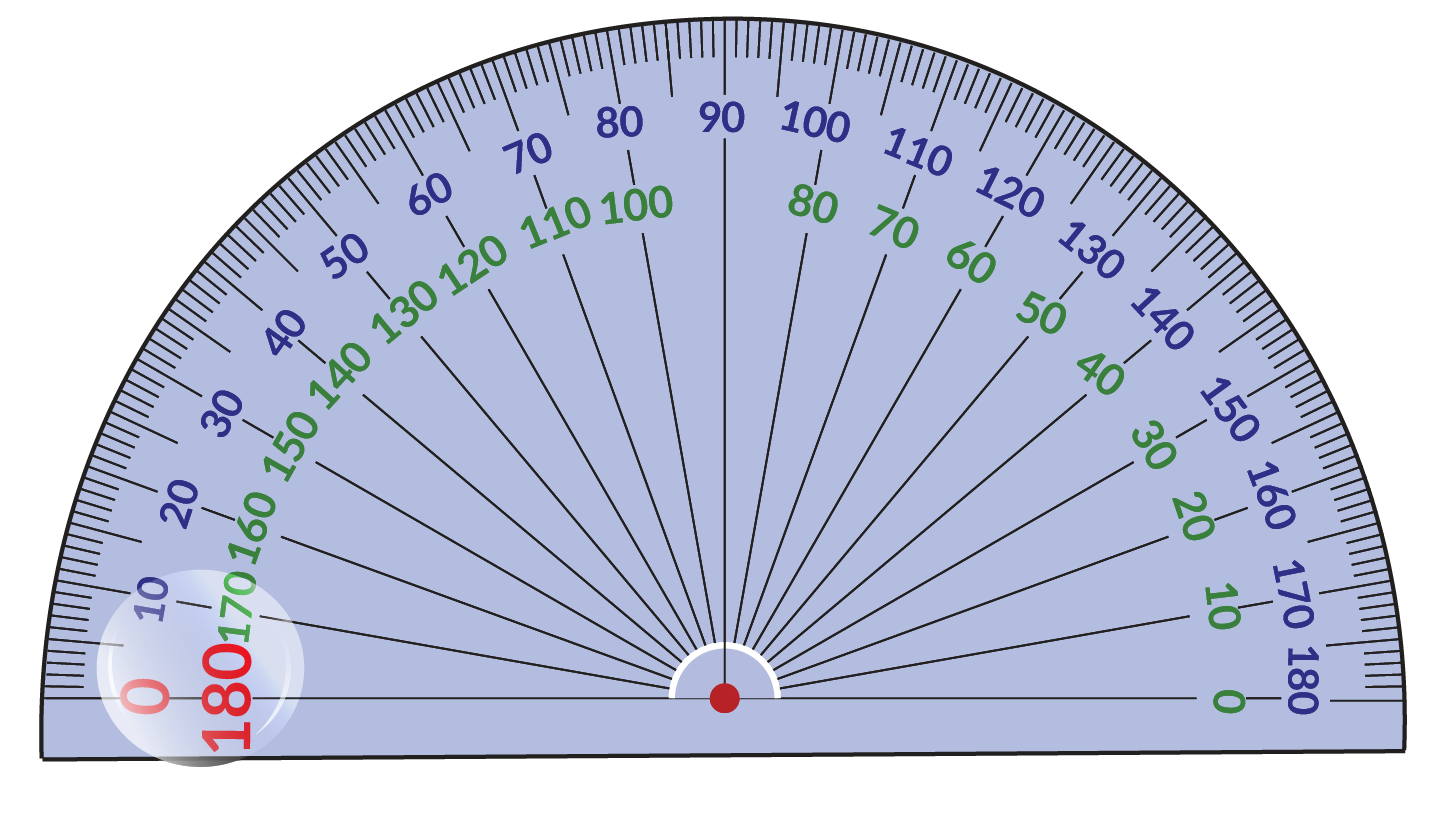The inner readings and outer reading supplements each other. i.e, they add up to  $$180^ \circ$$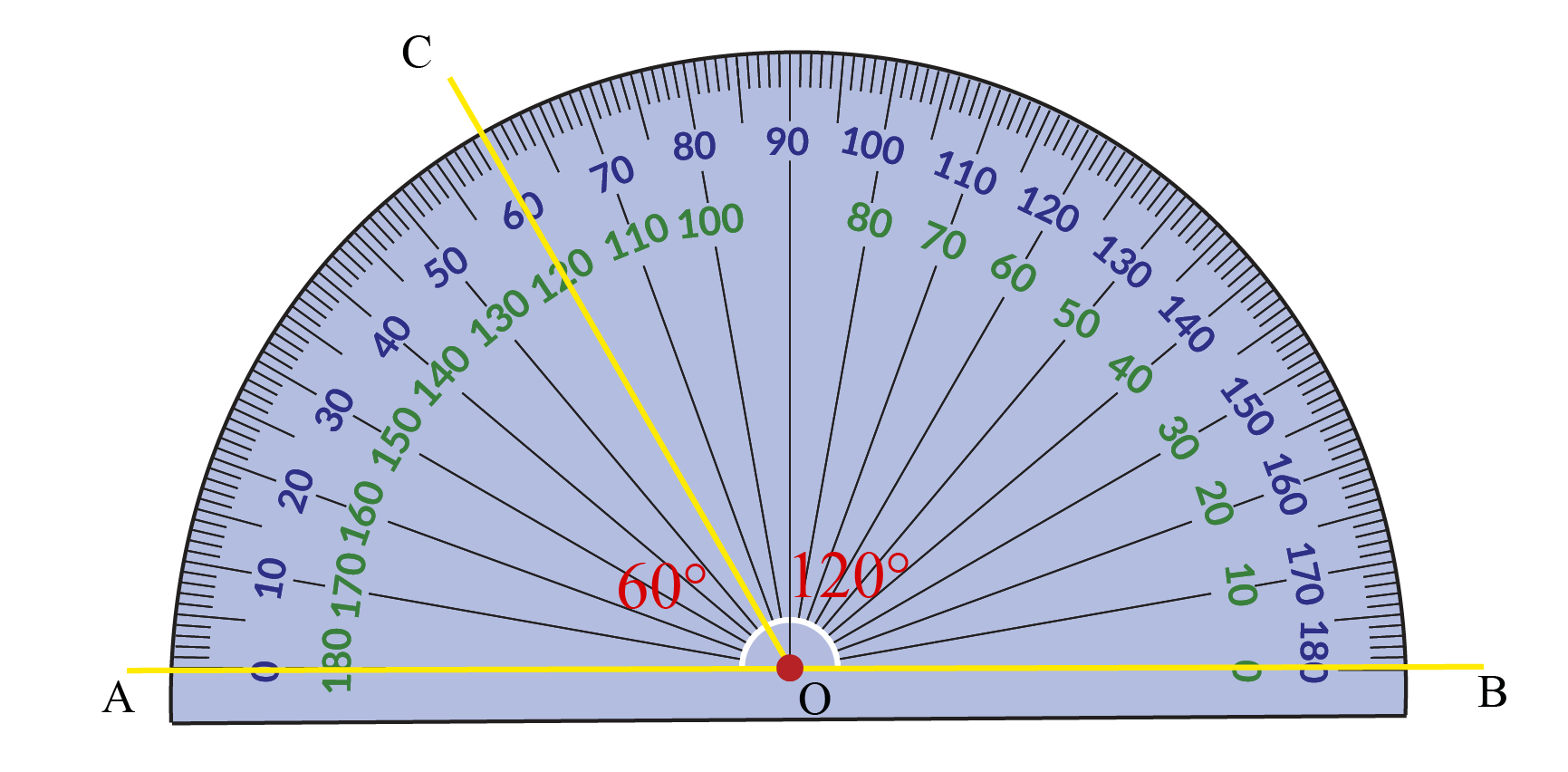If the angle to be measured is at the left part of the protractor, we need to use the outer reading of the protractor.

$$\angle \text{AOC} = 60^ \circ$$

If the angle to be measured is at the right part of the protractor, we need to use the inner readings of the protractor.

$$\angle \text{BOC} = 120^ \circ$$

## How to Use a Protractor?

An angle is formed between two rays.

Let us use the protractor and measure the angle $$\angle \text{COB}$$.

Align the baseline of the protractor with the line $$\text{AB}$$.

The center circle of the protractor at the vertex $$\text{O}$$.

Now, identify the reading on the protractor where Ray $$\text{C}$$ is extending.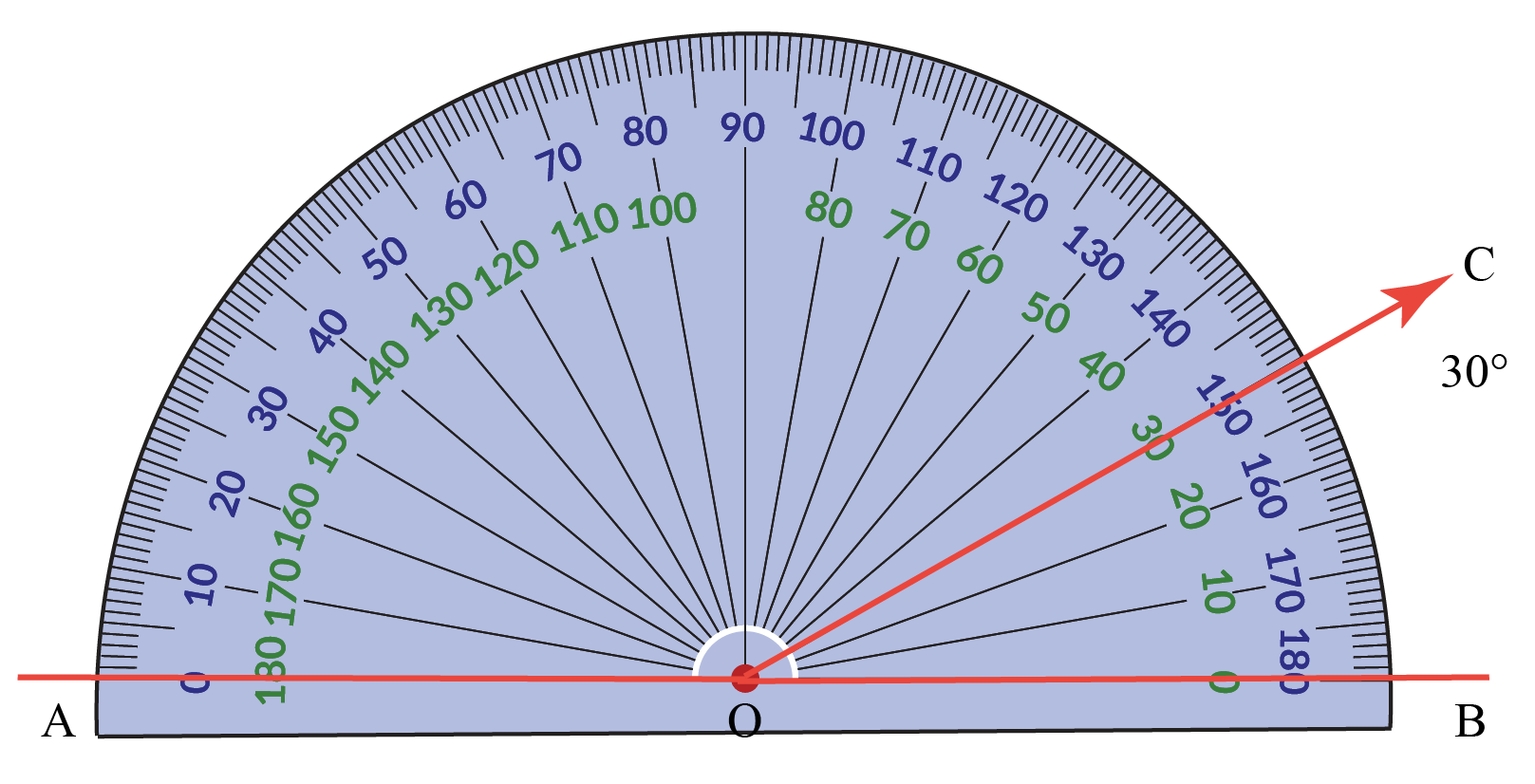$\angle \text{COB} = 30^\circ$

What do you think is the measure of $$\!\angle \text{AOC}$$?

If the angle to be measured is rotated or tilted, tilt your protractor to align with the base ray $$\text{OA}$$ and then measure.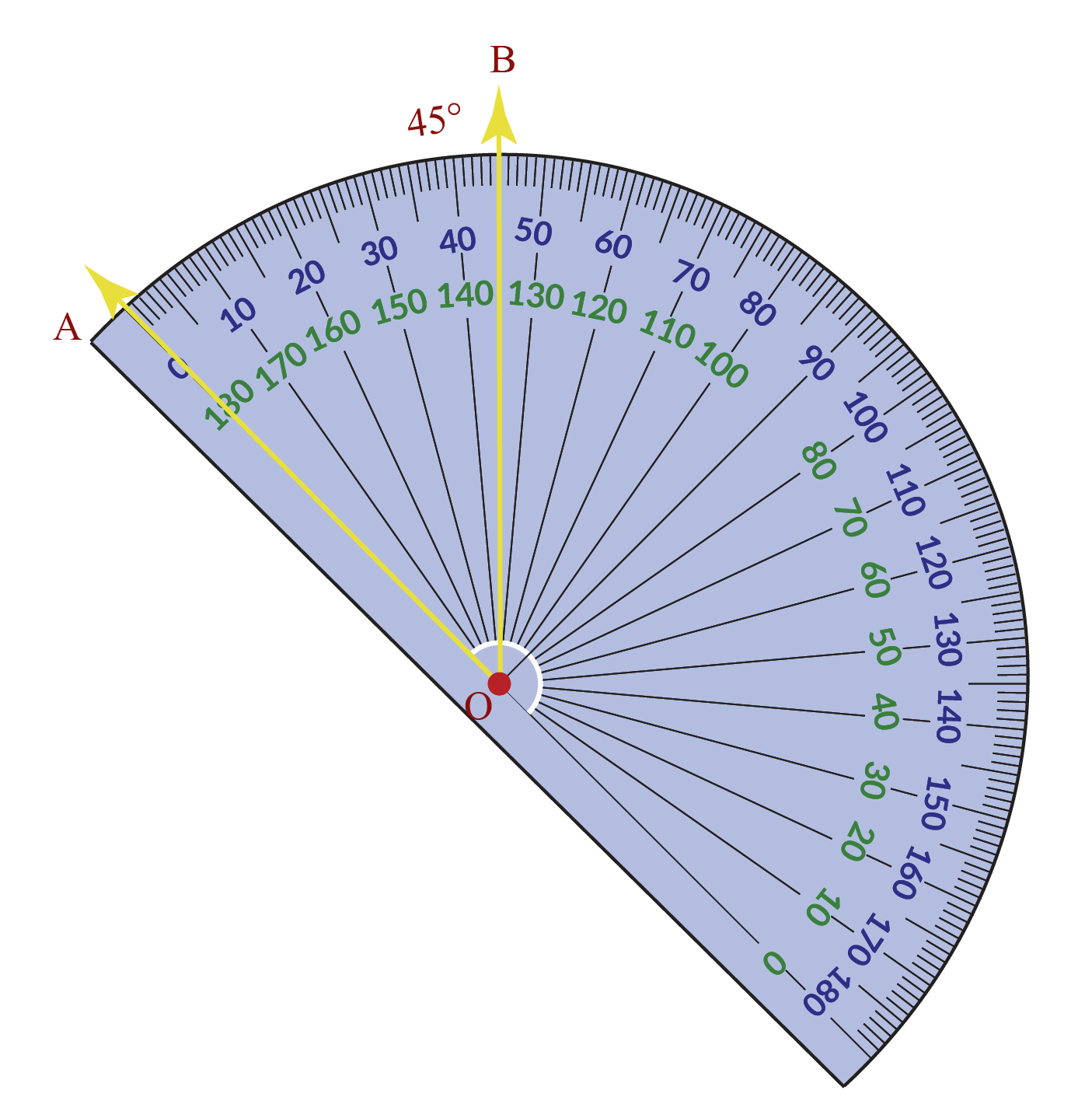In the above image,

$$\angle \text{AOB}\ = 45^ \circ$$

CLUEless in Math? Check out how CUEMATH Teachers will explain Protractor to your kid using interactive simulations & worksheets so they never have to memorise anything in Math again!

Explore Cuemath Live, Interactive & Personalised Online Classes to make your kid a Math Expert. Book a FREE trial class today!

## Measuring Angles from Protractor

The usage of a protractor in maths is considered essential as it helps measure angles.

In the previous section, we learnt how to use a protractor.

Here is an interactive simulation for you to measure different angles.

• Click on Create New Angle in the simulation given below.
• A new angle $$\angle \text{AOB}$$ will be generated.
• Using the protractor, measure $$\angle \text{AOB}$$ and enter the value in the space provided.

## Draw Angles with the Protractor

Let us see how to draw an angle of $$30 ^\circ$$ using a protractor.

• Draw a baseline $$\text{OA}$$
• Mark the point $$\text{O}$$ and place the center of the protractor at $$\text{O}$$.
• Align the baseline of the protractor with the line $$\text{OA}$$.
• In the outer readings, look for $$30 ^\circ$$ and mark it as point $$\text{B}$$
• Now using a scale, join $$\text{O}$$ and $$\text{B}$$

Look at the simulation below on how to draw a $$30 ^\circ$$ angle.

To measure $$220^\circ$$, a reflex angle, we can write it as $$180^\circ + 40^\circ$$

We can just flip the protractor along the baseline and then mark $$40^ \circ$$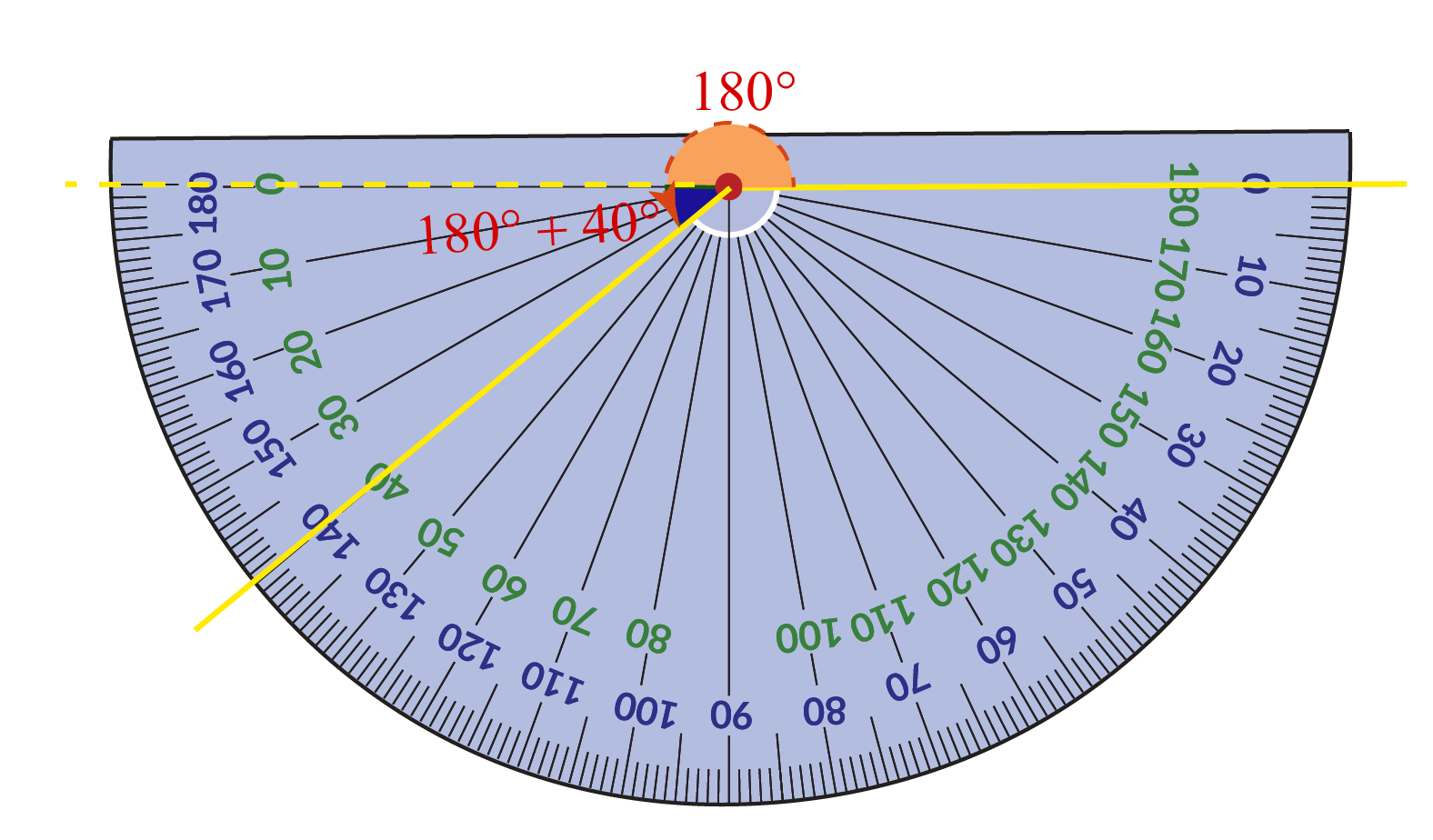$$180 ^\circ +40^\circ = 220^ \circ$$

We can also use a $$360^\circ$$ protractor to draw this angle.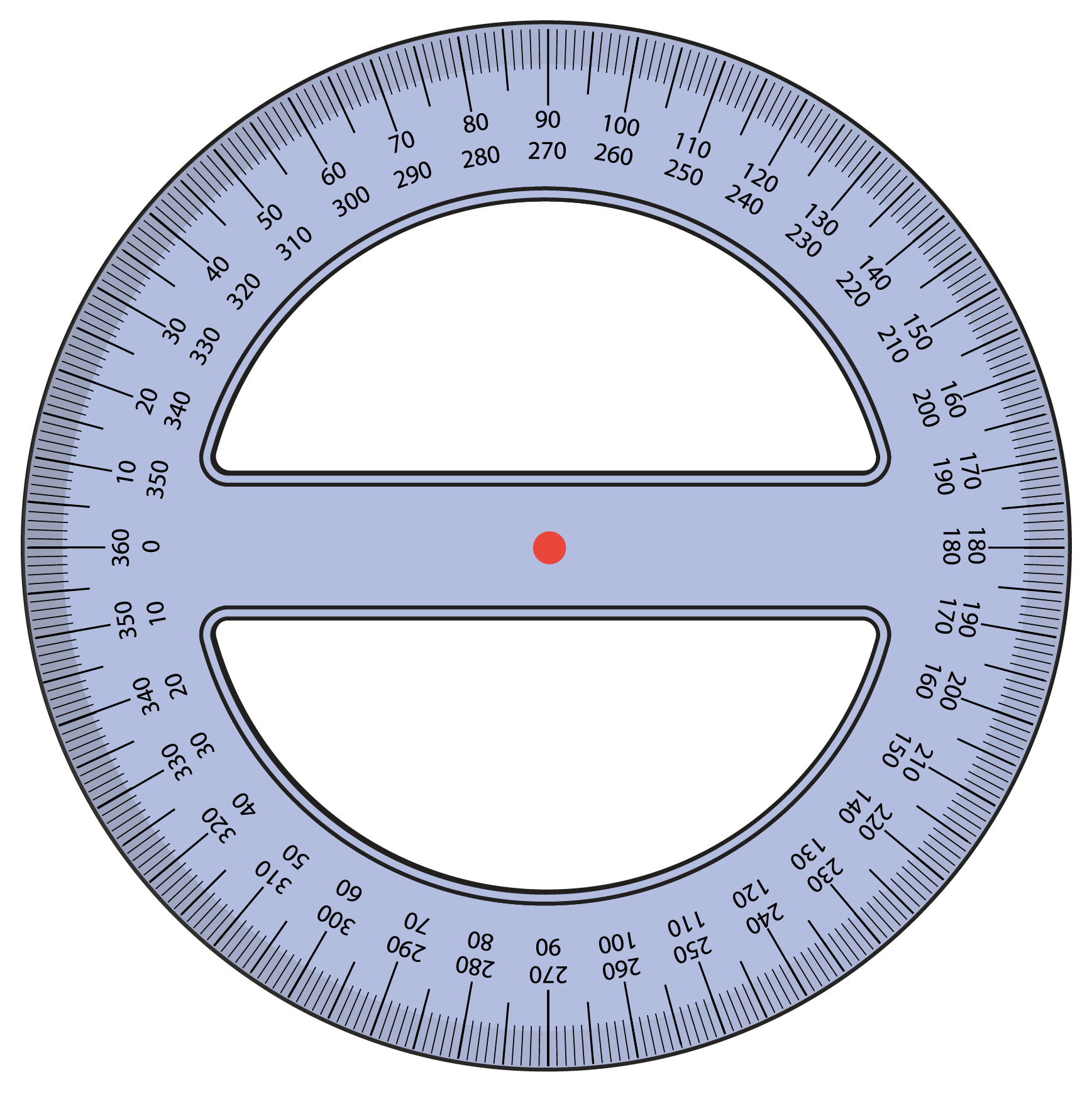The angle protractor tool and $$360^\circ$$ protractor gives more precise measurements.

More Important Topics
Numbers
Algebra
Geometry
Measurement
Money
Data
Trigonometry
Calculus